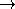## CGAL::Extremal_polygon_area_traits_2<K>advanced### Definition

The class Extremal_polygon_area_traits_2 provides the types and operations needed to compute a maximum area k-gon Pk that can be inscribed into a given convex polygon P using the function extremal_polygon_2.

### Requirements

The template parameter K is a model for Kernel.

### Is Model for the Concepts

ExtremalPolygonTraits_2

### Types

 Extremal_polygon_area_traits_2::FT typedef to K::FT. Extremal_polygon_area_traits_2::Point_2 typedef to K::Point_2. Extremal_polygon_area_traits_2::Less_xy_2 typedef to K::Less_xy_2. Extremal_polygon_area_traits_2::Orientation_2 typedef to K::Orientation_2. Extremal_polygon_area_traits_2::Operation AdaptableBinaryFunction class op: Point_2  × Point_2FT. For a fixed Point_2 root, op(p,q) returns twice the area of the triangle (root, q, p).

### Operations

int t.min_k () const returns 3.

FT t.init ( const Point_2& p, const Point_2& q) const
returns FT(0).

Operation t.operation ( const Point_2& p) const
returns Operation where p is the fixed root point.

template < class RandomAccessIterator, class OutputIterator >
OutputIterator
 t.compute_min_k_gon ( RandomAccessIterator points_begin, RandomAccessIterator points_end, FT& max_area, OutputIterator o)
const
writes the vertices of [points_begin, points_end) forming a maximum area triangle rooted at points_begin to o and returns the past-the-end iterator for that sequence (== o + 3).

Less_xy_2 t.less_xy_2_object ()
Orientation_2 t.orientation_2_object ()advanced